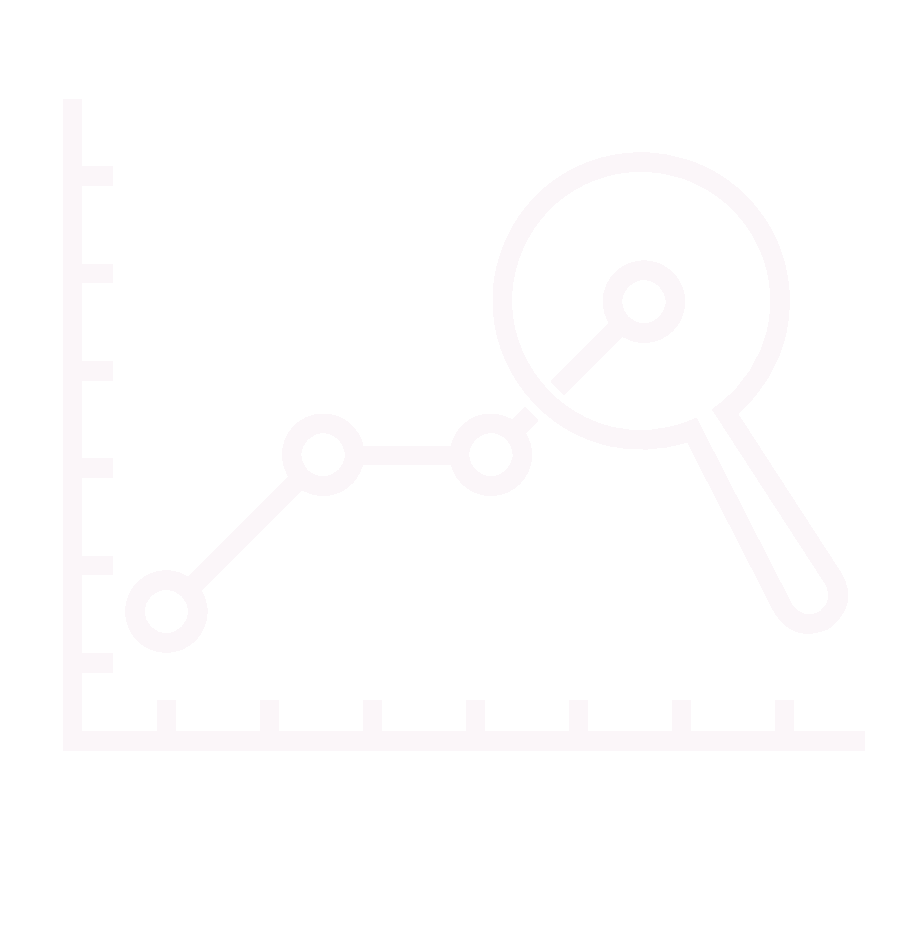Originally posted at http://www.howtomeasureanything.com, on Tuesday, February 03, 2009 9:20:28 AM, by lascar.

“Hello.

I’ve enjoyed the book and am trying to apply AIE to some of our IT decision making. Unfortunately I don’t have a statistician’s background and my college days are quite far away. So, [I’m] trying to catch up on some basics. Wikipedia is amazingly helpful in this sense.

I have two questions. If they are two basic for this forum, I’d appreciate anyone at least directing me to some resources which might help me answer them. Direct answers of cause are even more welcomed.

1. In Monte Carlo example in the book, the assumption is that most of the variables have Normal distribution. And if not, there are 2 more distributions mentioned – Uniform and Binary. I guess these are most common? My question is: how does one quickly evaluate what type of distribution is fitting for a variable? I’d guess it is quite straight forward with binary distribution. However from this article (http://en.wikipedia.org/wiki/List_of_probability_distributions), it seams there is quite a choice of distributions.

The MC scenario I’m running is to evaluate performance of a software package. I also realize that a quick proof of concept (running software and collecting metrics) might shed more light on distribution of some metric/variable. However that requires acquiring an expensive license first. So the decision I’m trying to facilitate is to prove that we need a POC and I need to calculate the value of improving on these measurements with POC’s help – alas – that cost of POC is worth lowering the uncertainty of measurements.

2. In the same Monte Carlo example standard deviation of 3.29 is used and the statement is that it is for 90% CI. However I’ve stumbled on this article (http://en.wikipedia.org/wiki/Standard_deviation#Rules_for_normally_distributed_data) and it seams the standard deviation for 90% is 1.645. 3.49 is closer to 99% CI. Can someone clarify, please?

Thank you.”

Thanks for your interest. First, yes there are quite a few distributions to choose from. I included the three simplest. The normal distribution is a very specific type of “bell curve”. I won’t go into how this bell curve is different from other bell curves, but the difference between this distribution and a uniform or binary is simple. The normal distribution is a range of values that are more likely in the middle but go out in both directions forever, albeit the odds are diminishingly small at the tails. The formula I gave for converting the bounds to normal distribution allows for values outside of your bounds – there is a 5% chance it could be higher than the upper bound and a 5% chance it is lower than the lower bound.

In a uniform distribution the values can only possible between the upper and lower bounds. Unlike the normal distribution, there is no chance the value could be outside of the bounds. Also, unlike the normal distribution, values are not more likely in the middle. Any value between the bounds of a uniform distribution is equally likely to any other value between the bounds. Use uniform distributions when you know that a variable can’t possibly be outside of the bounds. For example, if I know an uncertain variable about a productivity improvement from a new technology can’t be less than 0% and can’t be more than 10% (perhaps that’s the maximum amount of time spent on the activity being automated) then I would use a uniform distribution. However, if I’m not certain of those bounds but I think values around 5% are more likely than other values, then I might make it a normal distribution. Note that the normal, then, would allow the productivity improvement to be a negative value or greater than 10% even though values in the middle are more likely.

Binary is a simple one. It applies to events that either happen or do not. For example, if you are building a Monte Carlo simulation of a construction project and you want to model the chance of a labor strike, then you need to show that it will happen (or not) with a given probability. If there is a 10% chance of a strike then there is a 90% chance of no strike. The only values generated are either 1 or 0 and nothing in between. Of course, if there is a strike, you might want to use a normal or uniform to simulate the duration of the strike.

Regarding your second question, there is no inconsistency between the two values you mention. There are 3.29 standard deviations in a 90% CI if you subtract the upper bound from the lower bound, as I describe in the book. But there are 1.645 standard deviations from the middle of the range to either bound – which is half the distance between the bounds. (1.645 x 2 = 3.29) When you use 1.645, it is because you are starting with the middle value and computing the 90% CI. In the situation in the book, we start with a 90% CI and need to compute the standard deviation so we can simulate it.